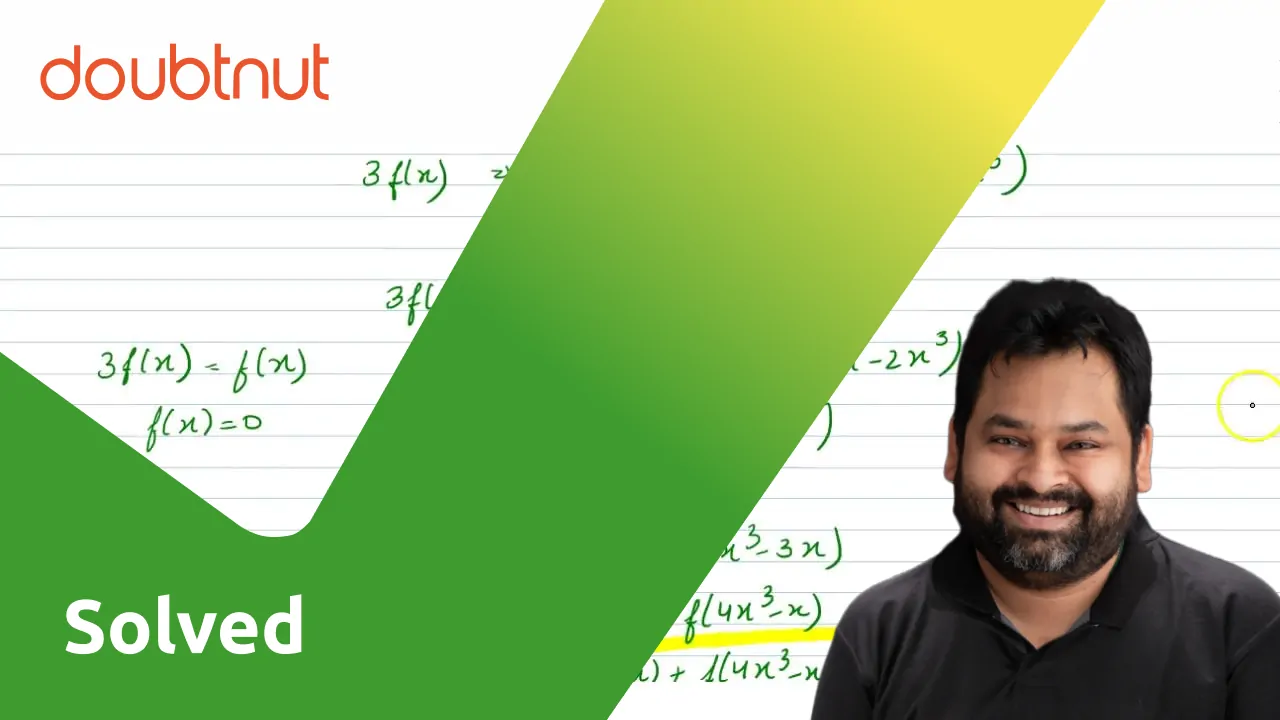Doubtnut App - Get 50 Lakh+ video solutions better than GoogleInstall Now

# Let f(x)+f(y)=f(x√1−y2+y√1−x2)[f(x) is not identically zero]. Then f(4x3−3x)+3f(x)=0 f(4x3−3x)=3f(x) f(2x√1−x2+2f(x)=0 f(2x√1−x2=2f(x)

Video Solution